Maths-
General
Easy

Question

# The number of ways in which mn students can be distributed equally among m sections is-

## (mn)n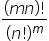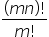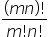Hint:

## The correct answer is:### Detailed SolutionIn this question, we have been asked to find the number of ways in which we can distribute mn students equally among m section. Now, we have been given that there are mn students in total and they have to be distributed equally among m sections.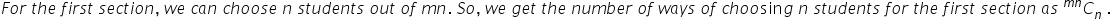For the second section, we will again choose n students but out of (mn – n) because n students have already been chosen for the first section. So, we get the number of ways of choosing n students for the second section as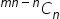Similarly, for the third section, we have to choose n students out of (mn – 2n). So, we get the number of ways of choosing n students for the third section as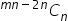And we will continue it in the same manner up to all mn students will not be divided into m section.So, for (m – 1)th section, we will choose n students from (mn – ( m – 2)n) student. So, we get the number of ways of choosing n students for (n – 1)th section, we get,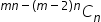And for the mth section, we get the number of ways for choosing students as,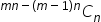Hence, we can write the total number of ways of distributing mn students in m section as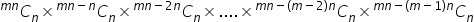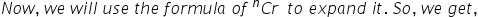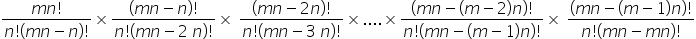And we can further write it as,While solving this question, the possible mistake one can make is by always choosing n students for all sections from mn students which is totally wrong because at a time one student can only be in 1 section. So, if n students are selected for 1 section then in the second section, we will choose from (mn – n).#### With Turito Foundation.#### Get an Expert Advice From Turito.